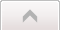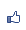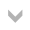Contents ...
udn網路城邦
TiKz 的label 與 pin
2015/04/21 10:05

label=[options] angle:text，如

\coodinate[label=A] (a) at (1,2) ;

label 的 options 通常用在node上面。而 angle 則是要標示的位置是在點的那個方位。如上方：

label=above:A或 label=90:A

absolute 則用以node的平均座標位置來標示：

\draw[help lines] (0,0) grid (5,5);

\node at (1,3) [rotate=-80, draw][label={[red,draw]right:label}]{main node};

\node at (3,3) [rotate=-80, draw][label={[red,draw, absolute]right:label}]{main node};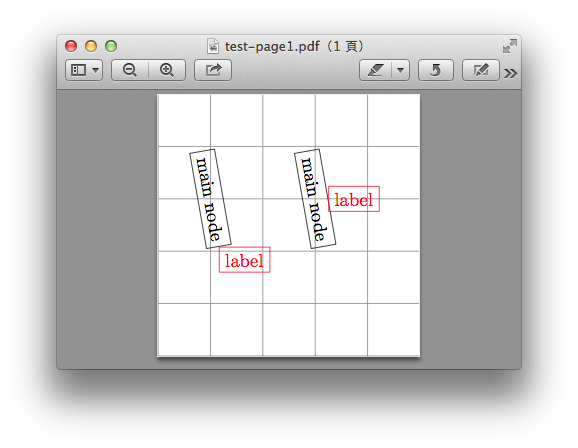label distance=長度，則是設定label 的距離：

\node at (1,1) [fill=blue!50,circle,inner sep=0.5 cm][label={[text=red]above:A}] [label={[text=brown,label distance=1cm]right:B}][pin={[pin distance=1.5cm, pin edge={thick}, inner sep=1pt]40:C}]{};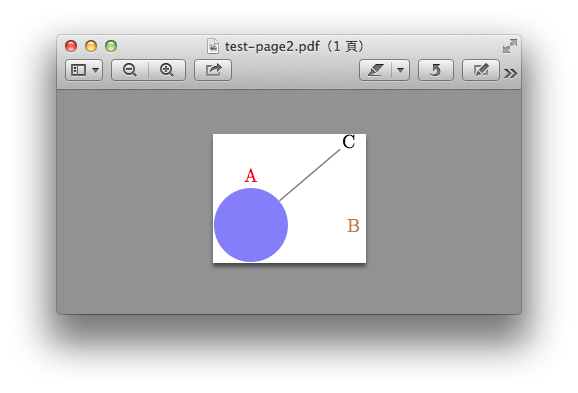pin的用法和label幾乎一樣：

pin=[options] angle: text

\usetikzlibrary{calc}

\node[inner color=white, outer color=orange, inner sep=0.5cm,circle, pin={[pin distance=4cm, pin edge=red, text=blue]130: Star}] at (2.5,2.5) {};

\draw[line width=2pt, draw=gray!80] (5,2.5) arc [start angle=0, end angle=360, x radius=2.5cm, y radius=2cm]node [name=earth, pos=0.6,ball color=blue!70,shading angle=-100, circle, pin={[pin distance=2cm,text=blue,pin edge=red, name=pinearth]left: Planet}]{};

\draw(\$(earth)+(0.5,0)\$) arc [start angle =0, end angle=360, x radius=0.5cm, y radius=0.3cm, dotted] node [circle,ball color=gray, shading angle=-120,inner sep=0.5mm,pos=0.2, name=moon, pin={[pin distance=3cm,text=blue, pin edge=red ]120: Satellite}]{};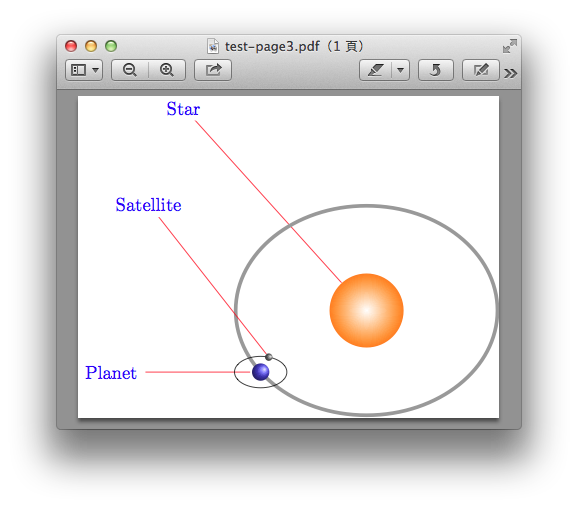\node (sun) at (3,3) {};

\node at (3,3)[name=sun]{};